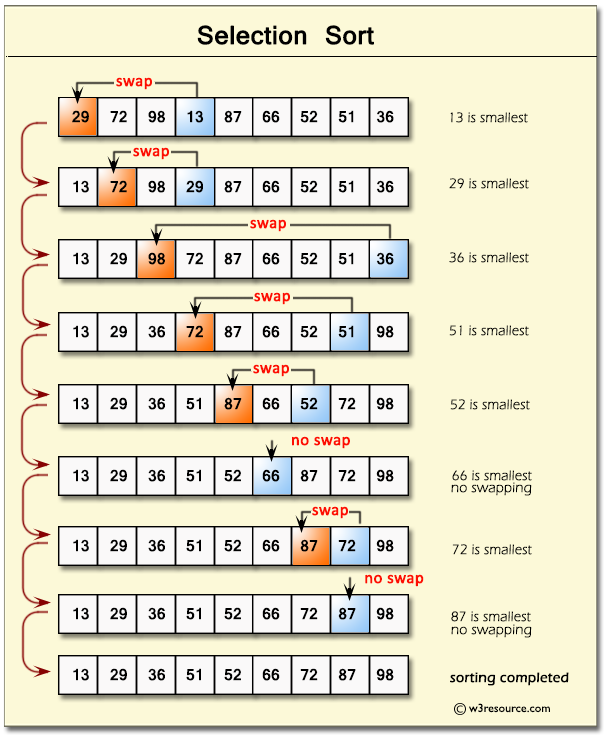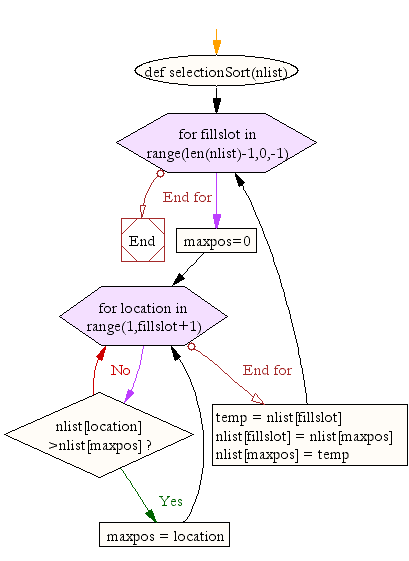﻿ Python Data Structures and Algorithms: Selection sort - w3resource# Python Data Structures and Algorithms: Selection sort

## Python Search and Sorting : Exercise-5 with Solution

Write a Python program to sort a list of elements using the selection sort algorithm.
Note : The selection sort improves on the bubble sort by making only one exchange for every pass through the list.

Pictorial Presentation : Selection SortSample Solution:-

Python Code:

``````def selectionSort(nlist):
for fillslot in range(len(nlist)-1,0,-1):
maxpos=0
for location in range(1,fillslot+1):
if nlist[location]>nlist[maxpos]:
maxpos = location

temp = nlist[fillslot]
nlist[fillslot] = nlist[maxpos]
nlist[maxpos] = temp

nlist = [14,46,43,27,57,41,45,21,70]
selectionSort(nlist)
print(nlist)
```
```

Sample Output:

```[14, 21, 27, 41, 43, 45, 46, 57, 70]
```

Flowchart:## Visualize Python code execution:

The following tool visualize what the computer is doing step-by-step as it executes the said program:

Python Code Editor :

What is the difficulty level of this exercise?

Test your Python skills with w3resource's quiz

﻿

## Python: Tips of the Day

Returns the maximum value of a list, after mapping each element to a value using the provided function

Example:

```def tips_max(lst, fn):
return max(map(fn, lst))

print(tips_max([{ 'n': 4 }, { 'n': 2 }, { 'n': 8 }, { 'n': 6 }], lambda v : v['n']))
```

Output:

```8
```